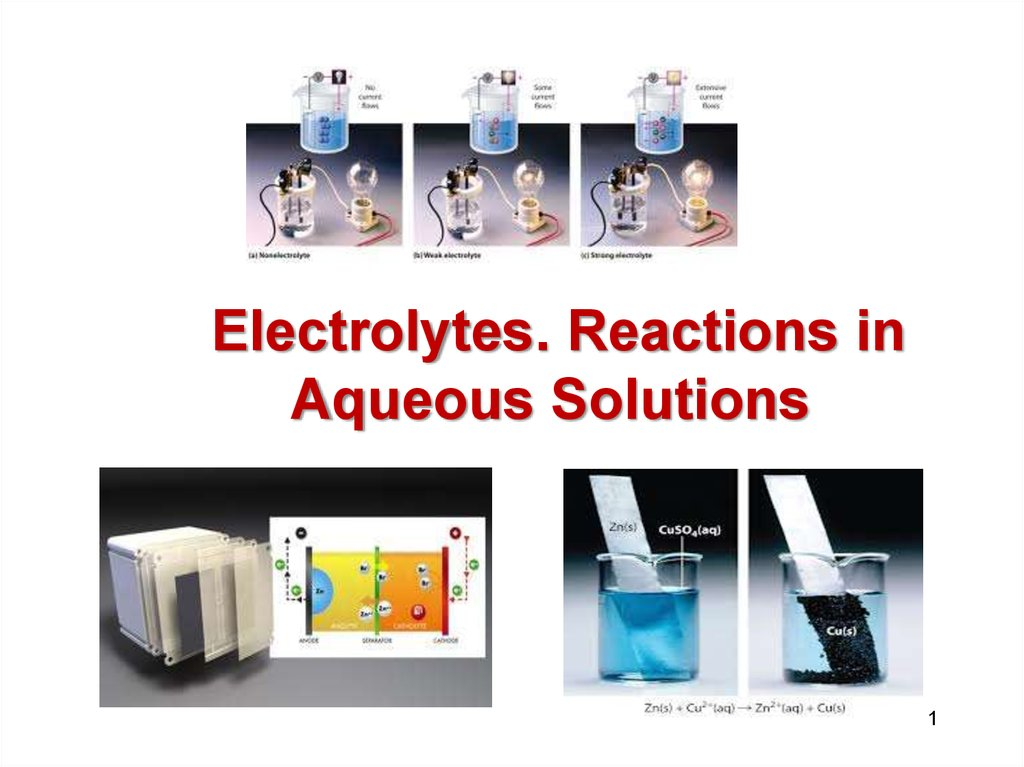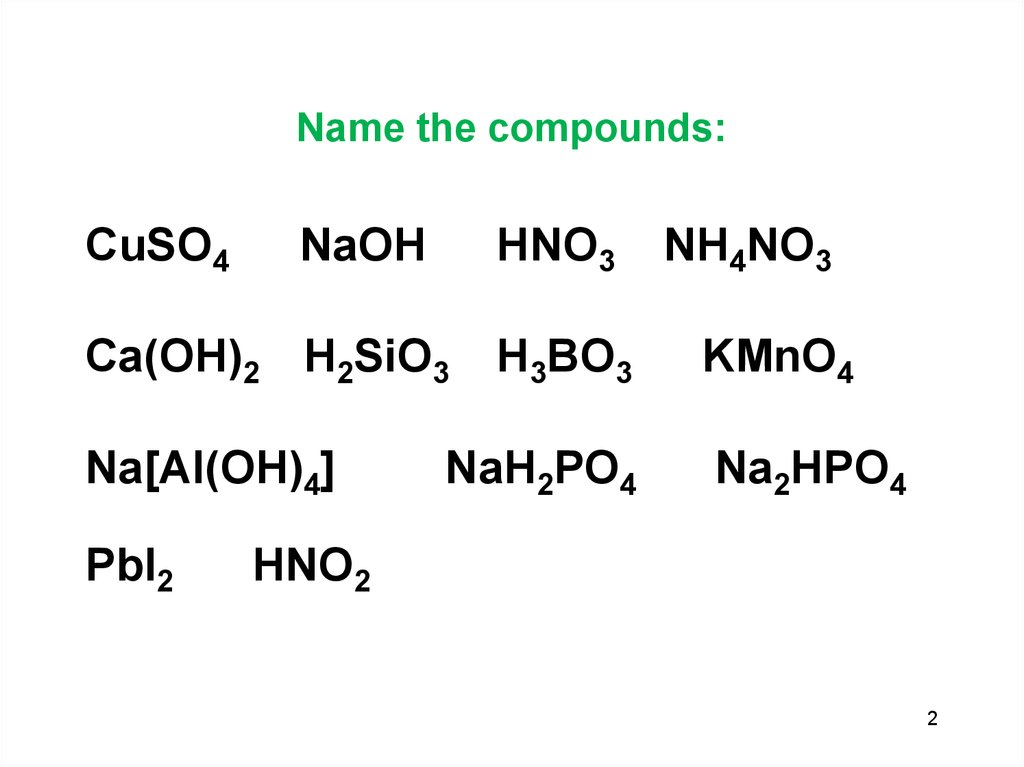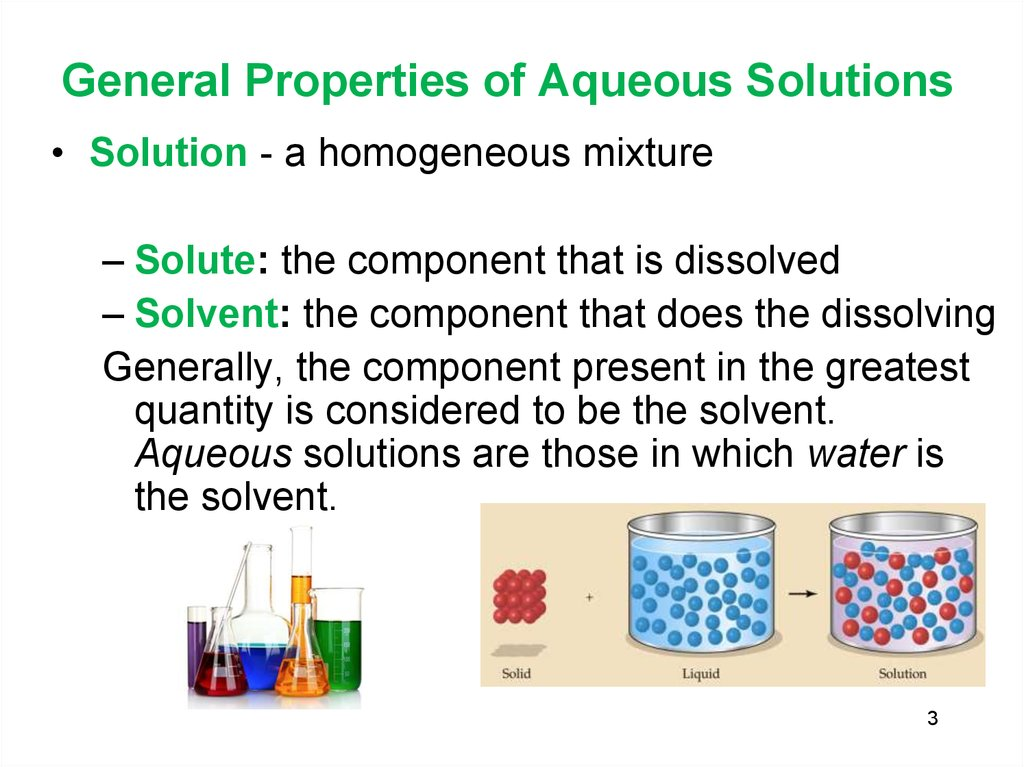# Electrolytes. Reactions in Aqueous Solutions

## 1.

Electrolytes. Reactions in
Aqueous Solutions
1

CuSO4
NaOH
HNO3
Ca(OH)2 H2SiO3
Na[Al(OH)4]
PbI2
H3BO3
NaH2PO4
NH4NO3
KMnO4
Na2HPO4
HNO2
2

## 3. General Properties of Aqueous Solutions

• Solution - a homogeneous mixture
– Solute: the component that is dissolved
– Solvent: the component that does the dissolving
Generally, the component present in the greatest
quantity is considered to be the solvent.
Aqueous solutions are those in which water is
the solvent.
3

## 4.

• Electrolytes and Nonelectrolytes
– Electrolyte: substance that, when dissolved
in water, produces a solution that conducts
electricity
• Contains ions
– Nonelectrolyte: substance that, when
dissolved in water, produces a solution that
does not conduct electricity
• Does not contain ions
4

## 5.

Dissociation - ionic compounds
separate into constituent ions
when dissolved in solution
Ionization - formation of ions by
molecular compounds when
dissolved
Arrhenius (1883))
5

## 6.

• Hydration: process by which water
molecules remove and surround
individual ions from the solid.

## 7. Acids and Bases

ACIDS
HAc → H+ (aq) + Ac- (aq)
ionization
HCl (g) → H+ (aq) + Cl- (aq)
HCl (g) → H+ (aq) + Cl- (aq)
hydronium ion
BASES
HCl (g) + H2O → H3O+ (aq) + Cl(aq)
H+(aq) + H2O → H3O+(aq)
MOH → M+ (aq) + OH- (aq)
NaOH (s) → Na+ (aq) + OH- (aq)

## 8.

Strong Electrolytes:
100% dissociation
• All water soluble ionic compounds,
strong acids and strong bases
• Conduct current very efficiently.
Weak electrolytes
• Partially ionized in solution
• Exist mostly as the molecular form
in solution
• Weak acids and weak bases
• Conduct only a small current.
8

## 9. Degree of dissociation

The fraction of total number of total number of
molecules of electrolytes dissolved, that ionizes at
equilibrium is known as degree of dissociation or
degree of ionization.
Degree of dissociation (DOD)
α=amount of substance of the reactant
dissociated/amount of substance of the reactant present
initially
In case of very strong acids and bases, degree of
dissociation will be close to 1. Less powerful acids and
bases will have lesser degree of dissociation.
9

## 10.

Method to Distinguish Types of Electrolytes
nonelectrolyte
weak electrolyte
strong electrolyte
10

11

## 12.

• Examples of weak electrolytes
– Weak acids
HC2H3O2(aq)
– Weak bases
NH3 (aq) + H2O(l)
C2H3O2 (aq) + H+ (aq)
NH4+ (aq) + OH (aq)
(Note: double arrows indicate a reaction that occurs in both
directions - a state of dynamic equilibrium exists)
Classify the following as nonelectrolyte, weak electrolyte or
strong electrolyte
H2SO4
CH3OH
H2CO3
12

## 13. Precipitation Reactions

• Precipitation (formation of a solid from two
aqueous solutions) occurs when product is
insoluble
• Produce insoluble ionic compounds
• Double replacement (or metathesis reaction)
• Solubility is the maximum amount of a solid
that can dissolve in a given amount of solvent
at a specified temperature
• Prediction based on solubility rules

## 14.

Simple Rules for Solubility
1. Most nitrate (NO3 ) salts are soluble.
2. Most alkali metals (group 1A) salts and NH4+ are soluble.
3. Most Cl , Br , and I salts are soluble
(NOT
Ag+, Pb2+, Hg22+)
4. Most sulfate salts are soluble (
NOT
BaSO4, PbSO4, HgSO4, CaSO4)
5. Most bases are only slightly soluble (NaOH, KOH are
soluble, Ba(OH)2, Ca(OH)2 are marginally soluble
6. Most S2 , CO32 , CrO42 , PO43 salts are only slightly
soluble.

## 15. Identify the Precipitate

(?)
(?)
Pb(NO3)2(aq) + 2NaI(aq) 2NaNO3(aq) + PbI2 (s)

## 16.

Mixing Solutions of Pb(NO3)2 and NaCl
16

## 17.

• Molecular equation: shows all compounds
represented by their chemical formulas
• Ionic equation: shows all strong electrolytes
as ions and all other substances (nonelectrolytes, weak electrolytes, gases) by
their chemical formulas

## 18.

Molecular equation:
Ionic equation:
• Net Ionic equation: shows only the reacting
species in the chemical equation
– Eliminates spectator ions
Net ionic equation:

## 19.

Steps in writing a net ionic equation
– Write the balanced molecular equation.
• Predict products by exchanging cations
and anions in reactants.
– Separate strong electrolytes into ions.
– Cancel spectator ions.
– Use the remaining species to write the net
ionic equation.
19

## 20.

Aqueous solutions of silver nitrate and sodium
sulfate are mixed. Write the net ionic reaction.
2AgNO3(aq)+Na2SO4(aq) 2NaNO3(?)+Ag2SO4(?)
All nitrates are soluble but silver sulfate is insoluble.
2Ag+(aq) + 2NO3 (aq) + 2Na+(aq) + SO42 (aq)
2Na+(aq) + 2NO3 (aq) + Ag2SO4(s)
Cancel spectators.
2Ag+(aq) + 2NO3 (aq) + 2Na+(aq) + SO42 (aq)
2Na+(aq) + 2NO3 (aq) + Ag2SO4(s)
Write the net ionic reaction.
2Ag+(aq) + SO42 (aq) Ag2SO4(s)
20

## 21. Aqueous Reactions and Chemical Analysis

Types of quantitative analysis
– Gravimetric analysis (mass analysis)
• Example: precipitation reaction
– Volumetric analysis (volume analysis)
• Example: titration
21

## 22.

Gravimetric Analysis
– One form: isolation of a precipitate
– Typical steps:
• Determine mass of unknown solid
• Dissolve unknown in water
• Combine with excess amount of known
substance to form a precipitate (excess
drives reaction to completion)
• Filter, dry and weigh the precipitate
• Use formula and mass of ppt to find % of
ion in unknown solid
22

## 23.

A 0.825 g sample of an ionic compound
containing chloride ions and an unknown
metal is dissolved in water and treated
with excess silver nitrate. If 1.725 g of
AgCl precipitate forms, what is the
percent by mass of Cl in the original
sample?
23

## 24.

Steps in solution:
• Find the % of Cl in AgCl
• Multiply the % of Cl by the mass of the
precipitate to obtain the Cl in the
sample
• Divide the mass of Cl in sample by
total mass of sample (multiply by 100
for %)
24

3
5
.
4
5
g
C
l
%
C
l
=
1
0
0
=
2
4
.
7
%
1
4
3
.
3
5
g
A
g
C
l
0
.
2
4
7
1
.
7
2
5
g
A
g
C
l
p
p
t
=
0
.
4
2
7
g
C
l
i
n
s
a
m
p
l
e
0
.
4
2
7
g
C
l
%
C
l
i
n
u
n
k
n
o
w
n
=
1
0
0
=
5
1
.
7
%
C
l
0
.
8
2
5
g
s
a
m
p
l
e
25

## 26.

• Volumetric analysis
– Commonly accomplished by titration
• Addition of a solution of known concentration
(standard solution) to another solution of
unknown concentration.
– Standardization is the determination of the
exact concentration of a solution.
– Equivalence point represents completion
of the reaction.
– Endpoint is where the titration is stopped.
– An indicator is used to signal the endpoint.
26

## 27.

Apparatus for Titration
27

## 28.

A student measured exactly 15.0 mL of
an unknown monoprotic acidic solution
and placed in an Erlenmeyer flask. An indicator
titration the student had used 35.0 mL of 0.12 M
NaOH to neutralize the acid. Calculate the
molarity of the acid.
0
.
1
2
m
o
l
N
a
O
H
1
m
o
l
a
c
i
d
0
.
0
3
5
L
N
a
O
H
=
0
.
0
0
4
2
m
o
l
a
c
i
d
1
L 1
m
o
l
b
a
s
e
0
.
0
0
4
2
m
o
l
M
=
=
0
.
2
8
M
a
c
i
d
0
.
0
1
5
L
28

## 29.

Calculate the molarity of 25.0 mL of a
monoprotic acid if it took 45.50 mL of 0.25 M
KOH to neutralize the acid.
0.25 mol KOH
1 mol acid
0.04550 L
0.01338 mol acid
L
1 mol KOH
0.01338 mol acid
0.455 M
0.0250 L
29